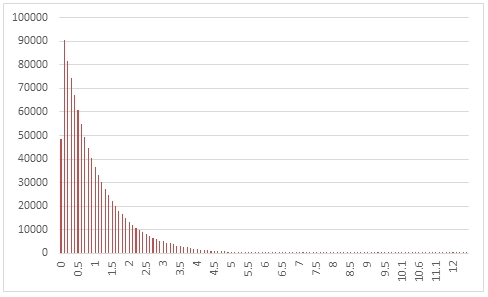# SQL Server random exponential distribution

RANDEXP

Updated: 31 March 2014

Use the table-valued function RANDEXP to generate a sequence of random numbers from an exponential distribution with rate @lambda.
Syntax
SELECT * FROM [wctMath].[wct].[RANDEXP](
<@Rows, int,>
,<@lambda, float,>)
Arguments
@Rows
the number of rows to generate. @Rows must be of the type int or of a type that implicitly converts to int.
@lambda
the rate parameter. @lambda must be of the type float or of a type that implicitly converts to float.
Return Types
RETURNS TABLE (
[Seq] [int] NULL,
[X] [float] NULL
)
Remarks
·         @lambda must be greater than zero.
·         If @lambda is NULL then @lambda is set to 1.
·         If @Rows is less than 1 then no rows are returned.
Examples
In this example we create a sequence 1,000,000 random numbers rounded to one decimal place from an exponential distribution with @lambda = 1, COUNT each result, paste then into Excel, and graph them.
SELECT
X,
COUNT(*) as [COUNT]
FROM (
SELECT
ROUND(X,1) as X
FROM
wct.RANDEXP(
1000000, --@Rows
1        --@lambda
)
)n
GROUP BY
X
ORDER BY
X

This produces the following result.In this example we generate 1,000,000 random numbers from an exponential distribution with @lambda of 2. We calculate the mean, standard deviation, skewness, and excess kurtosis from the resultant table and compare those values to the expected values for the distribution.
DECLARE @size as int = 1000000
DECLARE @mu as float = 2
DECLARE @mean as float = 1e+00/@mu
DECLARE @var as float = POWER(@mu,-2)
DECLARE @stdev as float = SQRT(@var)
DECLARE @skew as float = 2
DECLARE @kurt as float = 6

SELECT
stat,
[RANDEXP],
[EXPECTED]
FROM (
SELECT
x.*
FROM (
SELECT
AVG(x) as mean_EXP,
STDEVP(x) as stdev_EXP,
wct.SKEWNESS_P(x) as skew_EXP,
wct.KURTOSIS_P(x) as kurt_EXP
FROM
wct.RANDEXP(@size,@mu)
)n
CROSS APPLY(
VALUES
('RANDEXP','avg', mean_EXP),
('RANDEXP','stdev', stdev_EXP),
('RANDEXP','skew', skew_EXP),
('RANDEXP','kurt', kurt_EXP),
('EXPECTED','avg',@mean),
('EXPECTED','stdev',@stdev),
('EXPECTED','skew',@skew),
('EXPECTED','kurt',@kurt)
)x(fn_name,stat,val_stat)
)d
PIVOT(sum(val_stat) FOR fn_name in([RANDEXP],[EXPECTED])) P

This produces the following result (your result will be different).

 stat RANDEXP EXPECTED avg 0.500344868 0.5 kurt 5.918523715 6 skew 1.995981452 2 stdev 0.500702317 0.5

See Also

### PricingCopyright 2008-2021 Westclintech LLC         Privacy Policy        Terms of Service